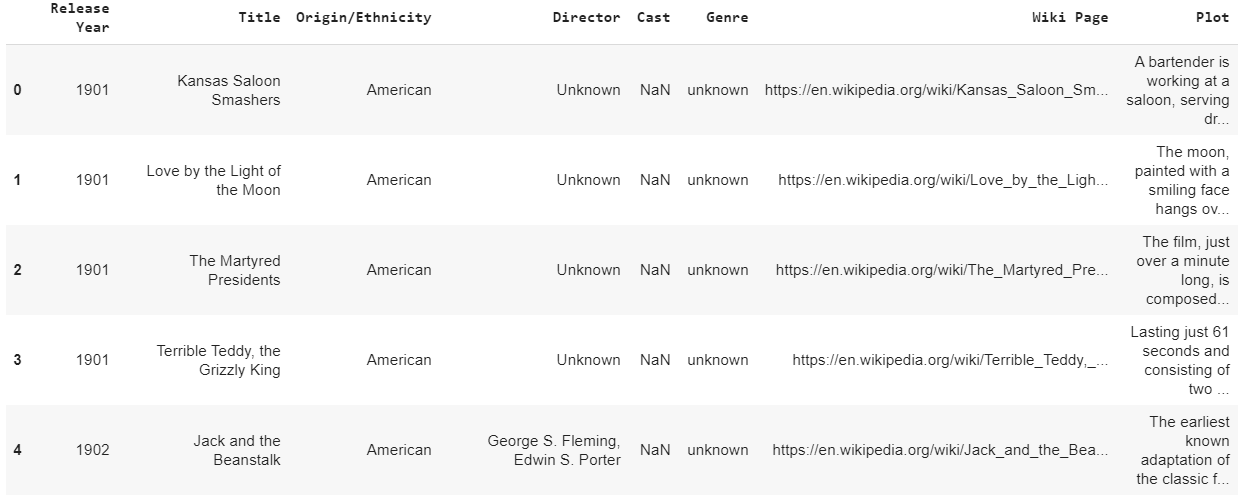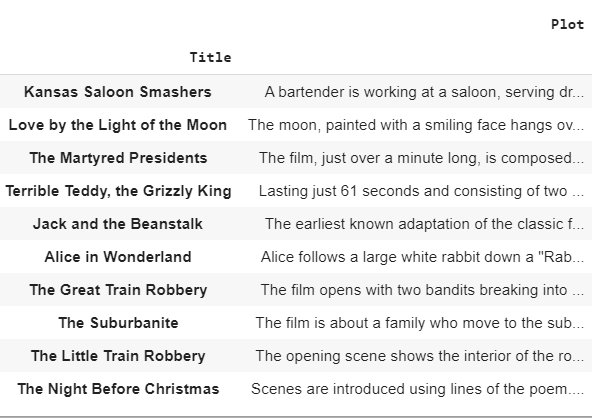# Movie recommender based on plot summary using TF-IDF Vectorization and Cosine similarity

Recommending movies to users can be done in multiple ways using content-based filtering and collaborative filtering approaches. Content-based filtering approach primarily focuses on the item similarity i.e., the similarity in movies, whereas collaborative filtering focuses on drawing a relation between different users of similar choices in watching movies.
Based on the plot of a movie that was watched by the user in the past, movies with a similar plot can be recommended to the user. This approach comes under content-based filtering as the recommendations are done only based on the user’s past activity.

Dataset used: A kaggle dataset which was scraped from wikipedia and contains plot summary of movies.

 `# Give the location of the dataset ` `path_dataset ``=``""  ` ` `  `import` `pandas as pd ` `data ``=` `pd.read_csv(path_dataset) ` `data.head() `

Output:There are movies that belong to different languages/origin in the dataset in varying numbers.

Code:

 `len``(data) ` ` `  `import` `numpy as np ` `np.unique(data[``'Origin / Ethnicity'``] ` ` `  `len``(data.loc[data[``'Origin / Ethnicity'``]``=``=``'American'``]) ` `len``(data.loc[data[``'Origin / Ethnicity'``]``=``=``'British'``]) `

Output:

```
34886    #Length of the dataset (Total number of rows/movies)

#Movies of various origins present in the dataset.
'Filipino', 'Hong Kong', 'Japanese', 'Kannada', 'Malayalam',
'Malaysian', 'Maldivian', 'Marathi', 'Punjabi', 'Russian',
'South_Korean', 'Tamil', 'Telugu', 'Turkish'], dtype=object)

17377    #Number of movies of American origin
3670     #Number of movies of British origin

```

Out of the different columns in the dataset, only the required columns are the movie name and the movie plot. Considering a subset of the above dataset, we use only American and British movies. The subset dataset consists of 21047 movies.

Code:

 `# Concatenating American and British movies ` `df1 ``=` `pd.DataFrame(data.loc[data[``'Origin / Ethnicity'``]``=``=``'American'``]) ` `df2 ``=` `pd.DataFrame(data.loc[data[``'Origin / Ethnicity'``]``=``=``'British'``]) ` `data ``=` `pd.concat([df1, df2], ignore_index ``=` `True``) ` ` `  `len``(data) ` ` `  `finaldata ``=` `data[[``"Title"``, ``"Plot"``]]          ``# Required columns - Title and movie plot ` `finaldata ``=` `finaldata.set_index(``'Title'``)    ``# Setting the movie title as index ` ` `  `finaldata.head(``10``) ` `finaldata[``"Plot"``][``0``] `

Output:

```
21047    #Number of rows in the new dataset

# First 10 rows of the new dataset#Plot of the first movie
A bartender is working at a saloon, serving drinks to customers. After he fills a stereotypically Irish man's bucket with beer, Carrie Nation and her followers burst inside. They assault the Irish man, pulling his hat over his eyes and then dumping the beer over his head. The group then begin wrecking the bar, smashing the fixtures, mirrors, and breaking the cash register. The bartender then sprays seltzer water in Nation's face before a group of policemen appear and order everybody to leave.

```

Code: Applying natural language processing techniques to pre-process the movie plots:

 `import` `nltk ` `nltk.download(``'punkt'``) ` `nltk.download(``'averaged_perceptron_tagger'``) ` `nltk.download(``'wordnet'``) ` ` `  ` `  `from` `nltk.stem ``import` `WordNetLemmatizer ` `lemmatizer ``=` `WordNetLemmatizer() ` ` `  `from` `nltk.corpus ``import` `stopwords ` `nltk.download(``'stopwords'``) ` `stop_words ``=` `set``(stopwords.words(``'english'``)) ` ` `  `VERB_CODES ``=` `{``'VB'``, ``'VBD'``, ``'VBG'``, ``'VBN'``, ``'VBP'``, ``'VBZ'``} `

Data pre-processing steps:

• Plot summary is converted into tokens, Using NLTK word tokenizer.
• Using NLTK POS tagger, POS tags of tokens are extracted.
• Lemmatization is considered better over stemming, because lemmatization does morphological analysis of words.
• Lemmatization is done by removing inflectional endings of tokens, through NLTK Word-net lemmatizer.
• Common words are removed to increase the importance of tokens. From NLTK library, English stop words are downloaded and removed from the movie plots.
• Few general contractions are replaced with original words.

Code:

 `def` `preprocess_sentences(text): ` `  ``text ``=` `text.lower() ` `  ``temp_sent ``=``[] ` `  ``words ``=` `nltk.word_tokenize(text) ` `  ``tags ``=` `nltk.pos_tag(words) ` `  ``for` `i, word ``in` `enumerate``(words): ` `      ``if` `tags[i][``1``] ``in` `VERB_CODES:  ` `          ``lemmatized ``=` `lemmatizer.lemmatize(word, ``'v'``) ` `      ``else``: ` `          ``lemmatized ``=` `lemmatizer.lemmatize(word) ` `      ``if` `lemmatized ``not` `in` `stop_words ``and` `lemmatized.isalpha(): ` `          ``temp_sent.append(lemmatized) ` `         `  `  ``finalsent ``=` `' '``.join(temp_sent) ` `  ``finalsent ``=` `finalsent.replace(``"n't"``, ``" not"``) ` `  ``finalsent ``=` `finalsent.replace(``"'m"``, ``" am"``) ` `  ``finalsent ``=` `finalsent.replace(``"'s"``, ``" is"``) ` `  ``finalsent ``=` `finalsent.replace(``"'re"``, ``" are"``) ` `  ``finalsent ``=` `finalsent.replace(``"'ll"``, ``" will"``) ` `  ``finalsent ``=` `finalsent.replace(``"'ve"``, ``" have"``) ` `  ``finalsent ``=` `finalsent.replace(``"'d"``, ``" would"``) ` `  ``return` `finalsent ` ` `  `finaldata[``"plot_processed"``]``=` `finaldata[``"Plot"``].``apply``(preprocess_sentences) ` `finaldata.head() `

TF-IDF (TERM FREQUENCY-INVERSE DOCUMENT FREQUENCY) Vectorization:

1. Term Frequency (TF):The number of times a word appears in a document divided by the total number of words in the document. Every document has its own term frequency.
2. Inverse Data Frequency (IDF): The log of the number of documents divided by the number of documents that contain the word. Inverse data frequency determines the weight of rare words across all documents in the corpus.

Scikit-Learn provides a transformer called the TfidfVectorizer in the module called feature_extraction.text for vectorizing with TF–IDF scores.

Cosine Similarity:
The movie plots are transformed as vectors in a geometric space. Therefore the angle between two vectors represents the closeness of those two vectors. Cosine similarity calculates similarity by measuring the cosine of the angle between two vectors.

Code:

 `from` `sklearn.feature_extraction.text ``import` `TfidfVectorizer ` ` `  `# Vectorizing pre-processed movie plots using TF-IDF ` `tfidfvec ``=` `TfidfVectorizer() ` `tfidf_movieid ``=` `tfidfvec.fit_transform((finaldata[``"plot_processed"``])) ` ` `  `# Finding cosine similarity between vectors ` `from` `sklearn.metrics.pairwise ``import` `cosine_similarity ` `cos_sim ``=` `cosine_similarity(tfidf_movieid, tfidf_movieid) `

Code: Building recommendation function which gives top 10 similar movies:

 `# Storing indices of the data ` `indices ``=` `pd.Series(finaldata.index) ` ` `  `def` `recommendations(title, cosine_sim ``=` `cos_sim): ` `    ``recommended_movies ``=` `[] ` `    ``index ``=` `indices[indices ``=``=` `title].index[``0``] ` `    ``similarity_scores ``=` `pd.Series(cosine_sim[index]).sort_values(ascending ``=` `False``) ` `    ``top_10_movies ``=` `list``(similarity_scores.iloc[``1``:``11``].index) ` `    ``for` `i ``in` `top_10_movies: ` `        ``recommended_movies.append(``list``(finaldata.index)[i]) ` `    ``return` `recommended_movies `

Code: Using the above function to get plot based recommendations:

 `recommendations(``"Harry Potter and the Chamber of Secrets"``) `

Output:

```
Recommendations for the movie "Harry Potter and the Chamber of Secrets"

["Harry Potter and the Sorcerer's Stone",
"Harry Potter and the Philosopher's Stone",
'Harry Potter and the Deathly Hallows: Part I',
'Harry Potter and the Deathly Hallows: Part 1',
'Harry Potter and the Half-Blood Prince',
'Harry Potter and the Deathly Hallows: Part II',
'Harry Potter and the Deathly Hallows: Part 2',
'Harry Potter and the Order of the Phoenix',
'Harry Potter and the Goblet of Fire',
'Harry Potter and the Prisoner of Azkaban']

```

Code:

 `recommendations(``"Ice Age"``) `

Output:

```
Recommendations for the movie "Ice Age"

['Ice Age: The Meltdown',
'Ice Age: Dawn of the Dinosaurs',
'The Wrong Man',
'Ice Age: Continental Drift',
'The Buttercup Chain',
'Ice Age: Collision Course',
'Runaway Train',
'Corrina, Corrina',
'Sid and Nancy',

```

Code:

 `recommendations(``"Blackmail"``) `

Output:

```Recommendations for the movie "Blackmail"

['Checkpoint',
'Odds Against Tomorrow',
'The Beast with Five Fingers',
'Fruitvale Station',
'The Exile',
'The Black Swan',
'Small Town Gay Bar',
'Eye of the Cat',
'Blown Away',
'Brenda Starr, Reporter']

```

My Personal Notes arrow_drop_upCheck out this Author's contributed articles.

If you like GeeksforGeeks and would like to contribute, you can also write an article using contribute.geeksforgeeks.org or mail your article to contribute@geeksforgeeks.org. See your article appearing on the GeeksforGeeks main page and help other Geeks.

Please Improve this article if you find anything incorrect by clicking on the "Improve Article" button below.

Article Tags :
Practice Tags :

Be the First to upvote.

Please write to us at contribute@geeksforgeeks.org to report any issue with the above content.